# Mathematics - Signals And Systems - Exercise on Convolution

in StemSocial5 days ago[Image1]

## Introduction

Hey it's a me again @drifter1!

Today we continue with my mathematics series about Signals and Systems in order to cover Exercise on Convolution.

So, without further ado, let's dive straight into it!

## Discrete-Time Convolution Example [Based on P4.2 from Ref1]

Let's determine the discrete-time convolution of the following two signals: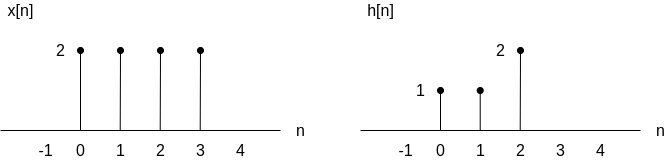### Solution

The convolution sum that we want to calculate is defined as: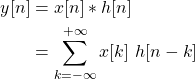Its easy to notice that the convolution sum requires an reflected version of h[n]. Reflecting h[n] about the origin and changing the unit from n to k leads to h[-k]: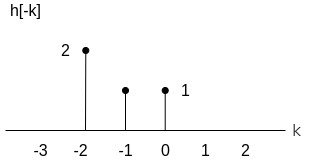which is basically h[n - k] for n = 0.

For the values n < 0 time-shifting leads to values of h[n - k] which result to zero contribution to the convolution sum. More specifically, the resulting h[n - k] are not in the closed range [0, 3] in which x[k] is non-zero. For example n = -1 and n = -2: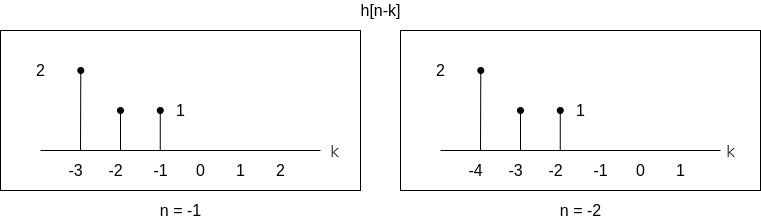As such, the sums that need to be calculated start at n = 0, which leads us to the question: "When do we stop?".

Let's start increasing n, which will of course lead to a shifting of h[n - k] to the right by 1, 2, 3 etc.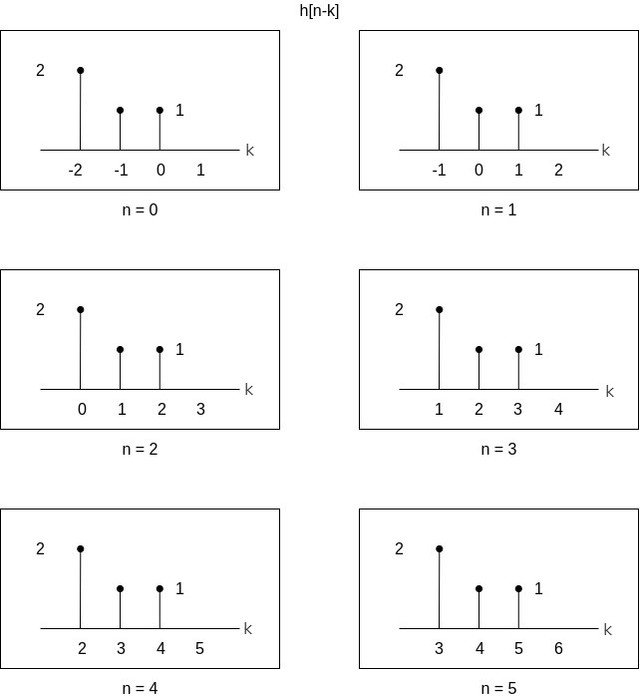After n = 5 the graph of h[n - k] again contributes zero to the convolution sum. Therefore, the convolution can be easily calculated by using n in the range [0, 5], which leads to a result, y[n], of length 6.

Generally, the result is equal to the sum of the lengths of the individual discrete-time signal sequences x[n] and h[n] minus 1: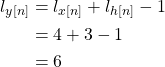So, how do we get the final result? Well, its simply multiplying x[k] by the various shifts of h[n - k] and summing up those values. The result of this sum is then the value of the convolution y[n] for each specific point in time.

The values n = 0 and n = 1 give us: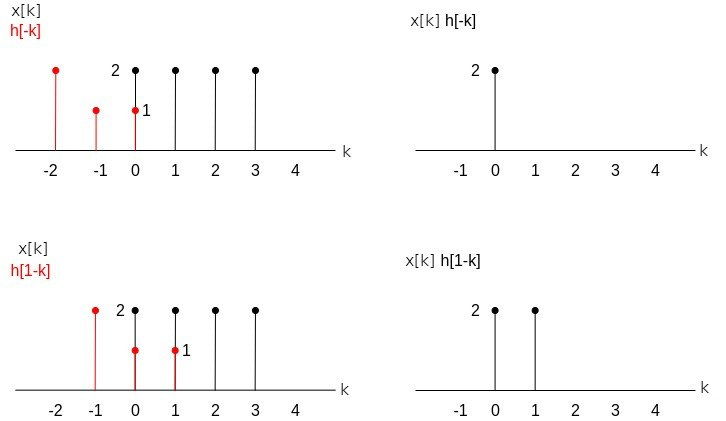which give us the values of the convolution y[n]: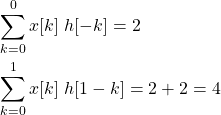Similarly, for the values n = 2 and n = 3 we have: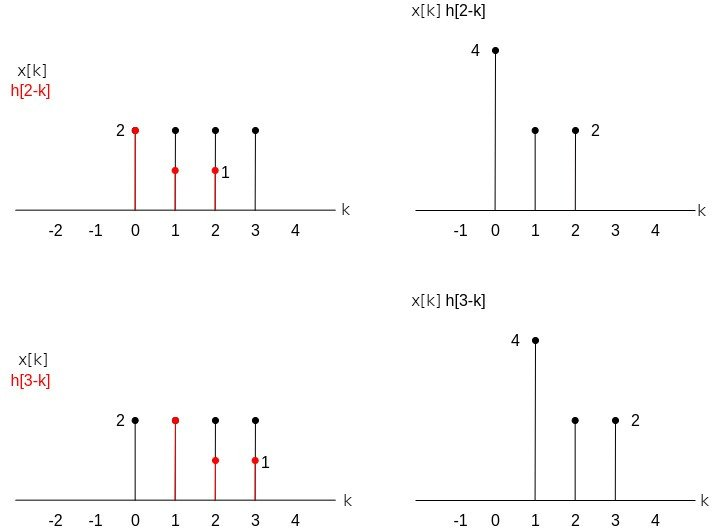which gives us two 8 's.

Lastly, for the values n = 4 and n = 5: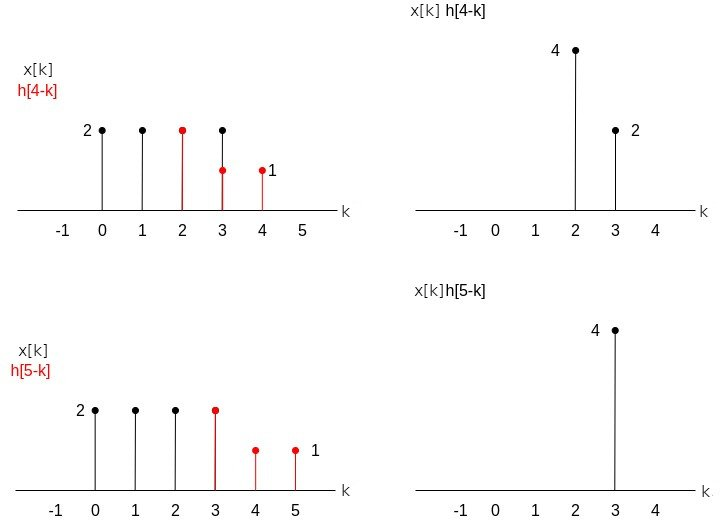and so the convolution contributions 6 and 4, respectively.

Finally, the convolution between x[n] and h[n], which is y[n], can be now visualized as follows: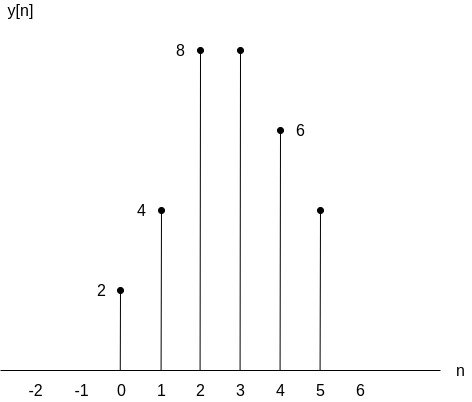## RESOURCES:

### Images

Mathematical equations used in this article were made using quicklatex.

Block diagrams and other visualizations were made using draw.io

## Final words | Next up

And this is actually it for today's post!

Next time we will get into exercises on other topics that we covered!

See Ya!Keep on drifting!